# RD Sharma Solutions - Chapter 22 - Mensuration - III (Part - 3), Class 8, Maths Notes | Study RD Sharma Solutions for Class 8 Mathematics - Class 8

## Class 8: RD Sharma Solutions - Chapter 22 - Mensuration - III (Part - 3), Class 8, Maths Notes | Study RD Sharma Solutions for Class 8 Mathematics - Class 8

The document RD Sharma Solutions - Chapter 22 - Mensuration - III (Part - 3), Class 8, Maths Notes | Study RD Sharma Solutions for Class 8 Mathematics - Class 8 is a part of the Class 8 Course RD Sharma Solutions for Class 8 Mathematics.
All you need of Class 8 at this link: Class 8

PAGE NO 22.26:

Question 20:

The trunk of a tree is cylindrical and its circumference is 176 cm. If the length of the trunk is 3 m, find the volume of the timber that can be obtained from the trunk.

Circumference of the tree = 176 cm = 2πr
Length of the trunk, h = 3 m = 300 cm
So, the radius (r) can be calculated by: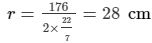Thus, the volume (V) of the timber can be calculated using the following formula:
V  =  πr2(h) = 22 (28 )2 (300) cm³  = 739200 cm³  = 0.74 m³

Question 21:

A well is dug 20 m deep and it has a diameter of 7 m. The earth which is so dug out is spread out on a rectangular plot 22 m long and 14 m broad. What is the height of the platform so formed?

Height of the well =  h m = 20 m
Diameter of the well  = d m = 7 m
Radius of the well =  r m = 3.5 m
Volume of the well = πr²h  = 22/7(3.5)2(20 ) m³ = 770 m³

Volume of the well = Volume of the rectangular plot
Length of the rectangular plot = 22 m
Breadth of the rectangular plot = 14 m
Volume of the rectangular plot = 770 m³  = (Length ×  Breadth  × Height) of the rectangular plot
Height  =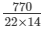= 2.5 m

Thus, the height of the platform is 2.5 m.

Question 22:

A well with 14 m diameter is dug 8 m deep. The earth taken out of it has been evenly spread all around it to a width of 21 m to form an embankment. Find the height of the embankment.

Diameter of the well =  d m = 14 m
Height of the well =  h m = 8 m
Radius of the well =  r m = 7 m
Volume of the well = πrh  = π(7 m)2(8 m) = 1232 m³
Volume of the well = Volume of the embankment

An embankment is a hollow cylinder with thickness. Its inner radius would be equal to the radius of the well, i.e. r  = 7 m, and its outer radius is R  = 7 + 21 = 28 m.

Volume of the embankment =  πh(R2-r2)

To find the height (h), we use the fact that the volume of the embankment is equal to the volume of the well.
1232 =  πh ((28)2-(7)2)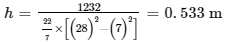Hence, the height of the embankment is 0.533 m or 53.3 cm.

Question 23:

A cylindrical container with diameter of base 56 cm contains sufficient water to submerge a rectangular solid of iron with dimensions 32 cm × 22 cm × 14 cm. Find the rise in the level of the water when the solid is completely submerged.

Diameter of the cylindrical container =  d cm = 56 cm
Radius of the cylindrical container =  r cm = 28 cm
Volume of cylindrical container = Volume of the rectangular solid
Length of the rectangular solid = 32 cm
Breadth of the rectangular solid = 22 cm
Height of the rectangular solid = 14 cm
Volume of the rectangular solid = Length x Breadth x Height = 32 cm x 22 cm x 14 cm = 9856 cm³
Volume of the cylindrical container = 9856 cm³  = πr²h
9856 cm³  =  22/7 (28 cm)2h

h  = 4 cm
Thus, when the solid is completely submerged, the water will rise up to 4 cm.

Question 24:

A rectangular sheet of paper 30 cm × 18 cm can be transformed into the curved surface of a right circular cylinder in two ways i.e., either by rolling the paper along its length or by rolling it along its breadth. Find the ratio of the volumes of the two cylinders thus formed.

Case 1:
Height =  h cm = 30 cm
Diameter =  d cm = 18 cm
Radius =  r cm = 9 cm
∴ Volume = (πr²h) or (π(9 )2(30)) = 2430π cm³

Case 2:
Height  =  h cm = 18 cm
Diameter =  d cm = 30 cm,
Radius =  r cm = 15 cm
∴ Volume = (πr²h) or (π(15 )2(18)) = 4050π cm³

Hence, the ratio of the volumes of the two cylinders formed is 3:5.

Question 25:

The rain which falls on a roof 18 m long and 16.5 m wide is allowed to be stored in a cylindrical tank 8 m in diameter. If it rains 10 cm on a day, what is the rise of water level in the tank due to it?

Length of the water on a roof = 18 m
Breadth of the water on a roof = 16.5 m
Height of the water on a roof = 10 cm = 0.1 m
Volume of the water on a roof = Length  ×  Breadth   ×  Height = 18 m ×  16.5 m  ×  0.1 m = 29.7 m³
Since water is to be stored in the cylindrical tank, the volume of water on a roof is equal to the volume of a cylindrical tank.
Volume of cylindrical tank = πr²h  = 29.7 m³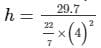= 0.5906 m = 59.06 cm
Thus, the rise of water level in the tank is 59.06 cm.

Question 26:

A piece of ductile metal is in the form of a cylinder of diameter 1 cm and length 5 cm. It is drawnout into a wire of diameter 1 mm. What will be the length of the wire so formed?

Diameter of the ductile metal = 1 cm
Radius of the ductile metal = 0.5 cm
Volume of the ductile metal = πr2(length) =  π(0.5 cm)2(5 cm) = 1.25π cm³
Ductile metal is drawn into a wire of diameter 1 mm.
Radius of the wire = 0.5 mm = 0.05 cm
Length of wire =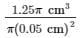= 500 cm  = 5 m
Thus, the length of wire is 5 m.

Question 27:

Find the length of 13.2 kg of copper wire of diameter 4 mm, when 1 cubic cm of copper weighs 8.4 gm.

Density of copper = Weight/Volume = 8.4 gram/1 cm³  = 8.4 gram/cm³
Volume = Weight/Density = 13.2 kg  ×  1000 gram/kg/8.4 gram/cm³  = 1571.43 cm³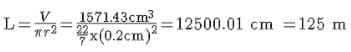Thus, length of 13.2 kg of copper is 125 m.

Question 28:

2.2 cubic dm of brass is to be drawn into a cylindrical wire 0.25 cm in diameter. Find the length of the wire.

Diameter of the cylindrical wire = 0.25 cm
Radius of the cylindrical wire = 0.125 cm
Volume of the brass = 2.2 dm³  = 2200 cm³

Volume of the brass = Volume of the cylindrical wire
Length of wire  =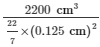= 44800 cm = 448 m
Thus, length of the wire is 448 m.

Question 29:

The difference between inside and outside surfaces of a cylindrical tube 14 cm long is 88 sq. cm. If the volume of the tube is 176 cubic cm, find the inner and outer radii of the tube.

r  = Inner radii of the tube
R  = Outer radii of the tube
h  = Length of the tube

2πh(R-r) = 88                          ... (1)
πh(R2-r2) = 176          ... (2)

Substituting h  = 14 cm in equation (1) and (2):

π(R-r) = 88/28             ... (1)
π(R-r)(R+r) = 176/14 ... (2)

Simplifying the second equation by substituting it with the first equation:
R+r = 4cm or R = (4−r)cm
Re-substituting R = 4−r into equation (1):
22/7 (4-r-r) =  88/28
4-2r  = 1
r  = 1.5 cm
R  = 4-1.5 = 2.5 cm

Hence, the inner and the outer radii of the tube are 1.5 and 2.5 cm, respectively.

Question 30:

Water flows out through a circular pipe whose internal diameter is 2 cm, at the rate of 6 metres per second into a cylindrical tank, the radius of whose base is 60 cm. Find the rise in the level of water in 30 minutes?

Radius of the circular pipe =  0.01 m
Length of the water column in 1 sec =  6 m
Volume of the water flowing in 1 s  = πr2 h = π(0.01)2(6) m³
Volume of the water flowing in 30 mins = π(0.01)2(6) × 30 × 60  m³
Let hm be the rise in the level of water in the cylindrical tank.
Volume of the cylindrical tank in which water is being flown = π(0.6)2 × h
Volume of water flowing in 30 mins = Volume of the cylindrical tank in which water is being flown
π(0.01)2(6) × 30 × 60  = π(0.6)2 × h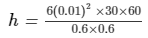h = 3 m

Question 31:

A cylindrical tube, open at both ends, is made of metal. The internal diameter of the tube is 10.4 cm and its length is 25 cm. The thickness of the metal is 8 mm everywhere. Calculate the volume of the metal.

Here, r  = Inner radius = 5.2 cm
t  = Thickness = 0.8 cm
h  = Length = 25 cm

R  =  r + t  = 5.2 cm + 0.8 cm = 6 cm
Volume of the metal =  π h (R2 - r2)
=  22/7 ×  (25) ×  ((6 )2 - (5.2 )2)
= 704 cm³
Thus, the volume of the metal is 704 cm³.

Question 32:

From a tap of inner radius 0.75 cm, water flows at the rate of 7 m per second. Find the volume in litres of water delivered by the pipe in one hour.

Radius of the water tap  =  0.75 cm  = 0.0075 m
Length of the water flowing in 1 s = 7 m = 700 cm
Volume of water flowing in 1s  = π(0.0075)2 × 700
Volume of the water flowing in 1 hour  = π(0.0075)2 × 700 × 60 × 60
Volume of the water flowing in 1 hour = 22/7× (0.0075)2 × 7 × 60 × 60 = 4.455 m³ = 4455l  (1000l = 1 m³)

Question 33:

A cylindrical water tank of diameter 1.4 m and height 2.1 m is being fed by a pipe of diameter 3.5 cm through which water flows at the rate of 2 metre per second. In how much time the tank will be filled?

Radius of the cylindrical tank  =  0.7 m
Height of the cylindrical tank  =  2.1 m
Volume of the cylindrical tank =  π(0.7)2(2.1) m³
Length of the water column flown from the pipe in 1 s =  2 m
Let the time taken to completely fill the water tank be x sec.
Length of the water column flown from the pipe in x sec =  2 x m
Radius of the pipe  =  1.75 cm =  0.0175 m
Volume of the water column flown from the pipe in x sec = π(0.0175)2 (2 x) m³
Volume of the cylindrical tank = Volume of the water column flown from the pipe
π(0.7)2(2.1) = π(0.0175)2 (2 x)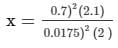= 1680 sec =  28 min

Thus, the time required to fill the water tank is 28 min.

Question 34:

A rectangular sheet of paper 30 cm × 18 cm can be transformed into the curved surface of a right circular cylinder in two ways i.e., either by rolling the paper along its length or by rolling it along its breadth. Find the ratio of the volumes of the two cylinders thus formed.

Let h cm be the length of the paper and r cm be the radius of the paper.
We know that the rectangular sheet of paper 30 cm x 18 cm can be transformed into two types of cylinder.
Type 1:
Length = 30 cm
Diameter = 18 cm
Volume = (πr²h) = (π(9cm)(30cm)) = 2430π cm³
Type 2:
Length = 18 cm
Diameter = 30 cm
Volume = (πr²h) or (π(15cm)(18cm)) = 4050π cm³
Hence, the ratio of the volumes of the two cylinders formed is 3:5.

Question 35:

How many litres of water flow out of a pipe having an area of cross-section of 5 cm² in one minute, if the speed of water in the pipe is 30 cm/sec?

We know:
Area of cross section = 5 cm²
Rate = 30 cm/s and
Time = 1 min
So, the volume of water flow is:
Volume = Volumetric rate  ×  Time = (30 cm/s)(5 cm²)(60 s/min) = 9000 cm³  = 9 litres

Thus, 9 litres of water flows out of the pipe.

Question 36:

A solid cylinder has a total surface area of 231 cm². Its curved surface area is 2/3 of the total surface area. Find the volume of the cylinder.

We know that the total surface area of the cylinder is 231 cm² and the curved surface area is 2/3 of the total surface area.
So, the curved surface area is:
2/3  ×  (231 cm²) = 154 cm²

Then, the radius of the cylinder can be calculated in the following manner:
Curved surface area = 2πrh
154 cm²  =  2πrh         ... (1)
Here, r  cm is the radius of the cylinder and h cm is the length of the cylinder.
2πr2  = (231-154) cm²  = 77 cm²
77 cm²  = 2πr2
From here, the radius (r) can be calculated in the following manner: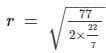r = 3.5 cm

Substituting this result into equation (1):
154 cm²  =  2π(3.5 cm)h
h =  154 cm² / (2x 22/7 x (3.5cm))
h  = 7 cm
∴ V  =  πr2h  =  22/7 x (3.5 cm)2 x (7 cm) = 269.5 cm³
Hence, the volume of the cylinder is 269.5 cm³.

The document RD Sharma Solutions - Chapter 22 - Mensuration - III (Part - 3), Class 8, Maths Notes | Study RD Sharma Solutions for Class 8 Mathematics - Class 8 is a part of the Class 8 Course RD Sharma Solutions for Class 8 Mathematics.
All you need of Class 8 at this link: Class 8Use Code STAYHOME200 and get INR 200 additional OFF

## RD Sharma Solutions for Class 8 Mathematics

88 docs

Track your progress, build streaks, highlight & save important lessons and more!

,

,

,

,

,

,

,

,

,

,

,

,

,

,

,

,

,

,

,

,

,

,

,

,

,

,

,

;